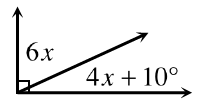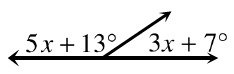Home > CC4 > Chapter 3 > Lesson 3.1.2 > Problem3-21

3-21.

For each diagram below, solve for x. Explain what angle properties or relationship(s) you used for each problem. Homework Help ✎

1.• The sum of the two angles is how many degrees?

1.• The sum of the two angles is how many degrees?